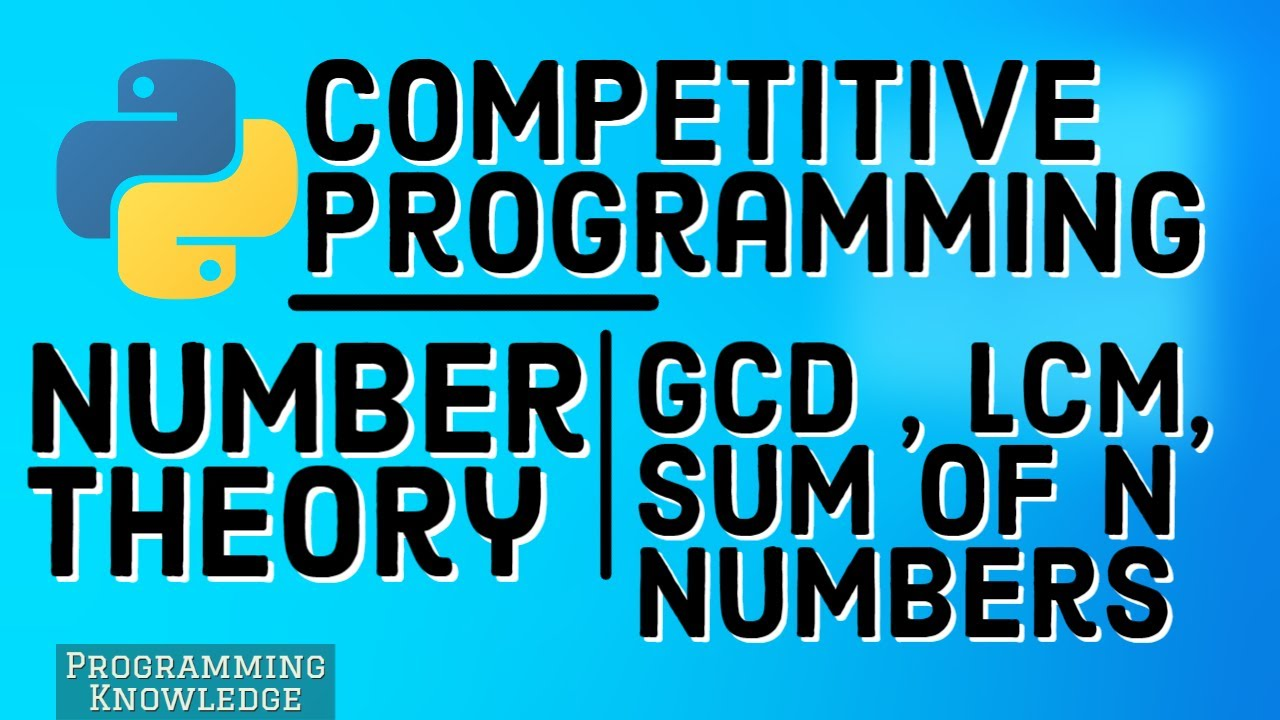1597235460

# Number Theory for Competitive Programming Using Python

Number Theory for Competitive Programming Using Python - Sum of n numbers, GCD and LCM
1.Sum upto n
2.Gcd
3.Lcm

#CompetitiveProgramming #ProgrammingKnowledge #NumberTheory #Python

#python #programming

## Buddha Community1619518440

## top 30 Python Tips and Tricks for Beginners

Welcome to my Blog , In this article, you are going to learn the top 10 python tips and tricks.

### 8) Check The Memory Usage Of An Object.

#python #python hacks tricks #python learning tips #python programming tricks #python tips #python tips and tricks #python tips and tricks advanced #python tips and tricks for beginners #python tips tricks and techniques #python tutorial #tips and tricks in python #tips to learn python #top 30 python tips and tricks for beginners1621499652

## Python Tips and Tricks for Competitive Programming

Python Programming language makes everything easier and straightforward. Effective use of its built-in libraries can save a lot of time and help with faster submissions while doing Competitive Programming. Below are few such useful tricks that every Pythonist should have at their fingertips:

• **Converting a number into a List of digits using map() Function: **

Below is the implementation to convert a given number into a list of digits:

#competitive programming #python programs #python-itertools #python-library #python-list #python-list-of-lists #python-map1619510796

## Lambda, Map, Filter functions in python

Welcome to my Blog, In this article, we will learn python lambda function, Map function, and filter function.

Lambda function in python: Lambda is a one line anonymous function and lambda takes any number of arguments but can only have one expression and python lambda syntax is

Syntax: x = lambda arguments : expression

Now i will show you some python lambda function examples:

#python #anonymous function python #filter function in python #lambda #lambda python 3 #map python #python filter #python filter lambda #python lambda #python lambda examples #python map1619607900

## Perfect Number Program In Python: How to check if a number is perfect or not?

Introduction

A number is said to be the perfect number if the sum of its proper divisors (not including the number itself) is equal to the number.

To get a better idea let’s consider an example, proper divisors of 6 are 1, 2, 3. Now the sum of these divisors is equal to 6 (1+2+3=6), so 6 is said to be a perfect number. Whereas if we consider another number like 12, proper divisors of 12 are 1, 2, 3, 4, 6. Now the sum of these divisors is not equal to 12, so 12 is not a perfect number.

Programming in Python is relatively simpler and more fun when compared to other languages because of its simpler syntax, good readability. Now that we are clear with the concept of perfect number let’s write a python program to check if a number is a perfect number or not. Let’s build a python code for checking if the given user input is a perfect number or not and explore the fun in coding with python.

#data science #how to check if a number is perfect #perfect number #perfect number in python #perfect number program in python #python1620418260

## Perfect Number Program In Python: How to check if a number is perfect or not?

Introduction

A number is said to be the perfect number if the sum of its proper divisors (not including the number itself) is equal to the number.

To get a better idea let’s consider an example, proper divisors of 6 are 1, 2, 3. Now the sum of these divisors is equal to 6 (1+2+3=6), so 6 is said to be a perfect number. Whereas if we consider another number like 12, proper divisors of 12 are 1, 2, 3, 4, 6. Now the sum of these divisors is not equal to 12, so 12 is not a perfect number.

Programming in Python is relatively simpler and more fun when compared to other languages because of its simpler syntax, good readability. Now that we are clear with the concept of perfect number let’s write a python program to check if a number is a perfect number or not. Let’s build a python code for checking if the given user input is a perfect number or not and explore the fun in coding with python.

#data science #how to check if a number is perfect #perfect number #perfect number in python #perfect number program in python #python